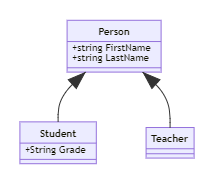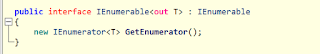# Covariance and Contravariance in Generics C# With Example

In this article, I’ll explain what covariance and contravariance are in C#. This feature was introduced in C# 4.0 as Covariance and Contravariance. This is an academic topic, but I’ll give you a description to easily relate to the code.

As per the Microsoft

In C#, covariance and Contravariance enable implicit reference conversion for array types, delegate types, and generic type arguments. Covariance preserves assignment compatibility, and Contravariance reverses it.To understand Covariance and Contravariance, we must first understand two terms: Less Derived and More Derived. As shown in the above class diagram, there are three classes: `Person`, `Student`, and `Teacher` (object is not shown in the image).

• The object is the ancestor of all types; it is always the ==Less Derived == type than other types.
• Person type is More Derived according to the Object type.
• The Student and Teacher type is More Derived according to Object and `Person` types.
Now let’s define covariance and Contravariance.\$ads={1}

## Covariance

When more derived types are assigned for less derived types, covariance is called. As you can see in the above graph that the student and the teacher is derived (subtype), so it is possible to use covariance because `IEnumerable` is covariance (Any generic type in C# with a parameter of type out behave as covariance)

``````	IEnumerable<Student> students = new List<Student>();
IEnumerable<Person> teachers = students;
``````## Contravariance

When less drive type is assigned to a more derived type, it is called contravariance. As you can see from the diagram above  `Student` and `Teacher` is derived (subtype) more so that the following code is legal because the type is contravariance in C#. (Any generic type in C# can be used as contravariance with the in parameter)

``````
Action<Person> printToConsole = (target) =>
{
Console.WriteLine(\$"{target.FirstNmae},{target.LastName}");
};
Action<Student> print = printToConsole;
print(new Student("John", "Doe", "IV"));

````````````public class Person
{

public string FirstNmae { get; set; }
public string LastName { get; set; }
public Person(string firstName, string lastName)
{
FirstNmae = firstName;
LastName = lastName;
}
public Person()
{

}
}

public class Teacher : Person
{

public Teacher(string FirstName, string LastName) : base(FirstName, LastName)
{

}
}
public class Student : Person
{

public string Grade { get; set; }
public Student(string FirstName, string LastName, string grade) : base(FirstName, LastName)
{
}
}
``````
``````public class PersonComparer : IComparer<Person>
{
public int Compare(Person x, Person y)
{
return x.FirstNmae.CompareTo(y.FirstNmae);
}
}
``````

## Contravariance Real World Example

``````
static void Main()
{

IComparer<Person> personComparer = new PersonComparer();
IComparer<Student> studentComparer = personComparer;
var students = new List<Student>(){
new Student("John","Doe","11"),
new Student("Julie","Doe","12"),
new Student("Bill","Gates","10"),
new Student("Aron","Sane","13")
};
students.Sort(studentComparer);
Console.WriteLine(students);

}
``````

## Covariance Real World Example

``````public static void Print(IEnumerable<Person> students){

foreach (var student in students)
{
Console.WriteLine(\$"{student.FirstNmae}-{student.LastName}");
}
}
``````
``````
static void Main()
{

IEnumerable<Student> students = new List<Student>(){
new Student("John","Doe","11"),
new Student("Julie","Doe","12"),
new Student("Bill","Gates","10"),
new Student("Aron","Sane","13")
};
Print(students);

}
``````

When the same type is assigned to same type then it is called Invariance.{alertSuccess}

Please do not post any spam link in the comment box😊